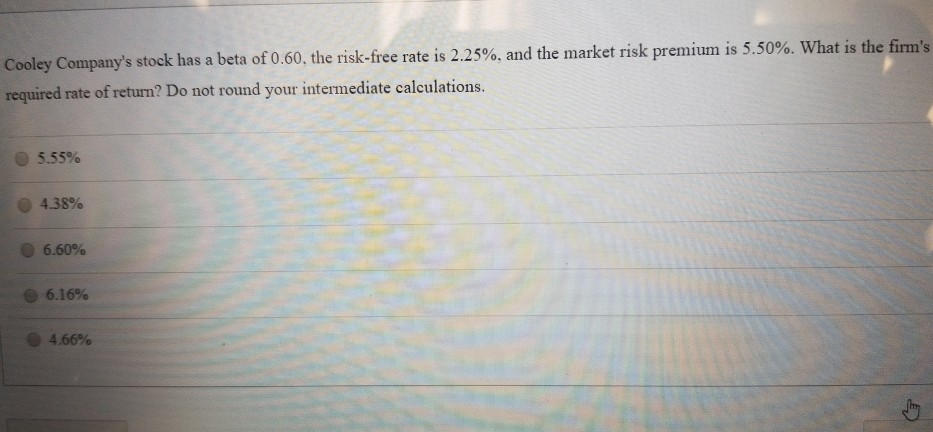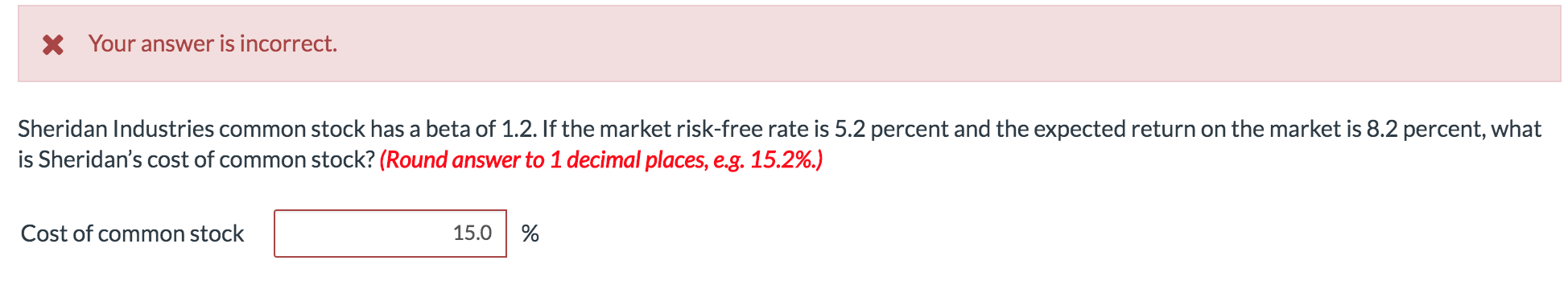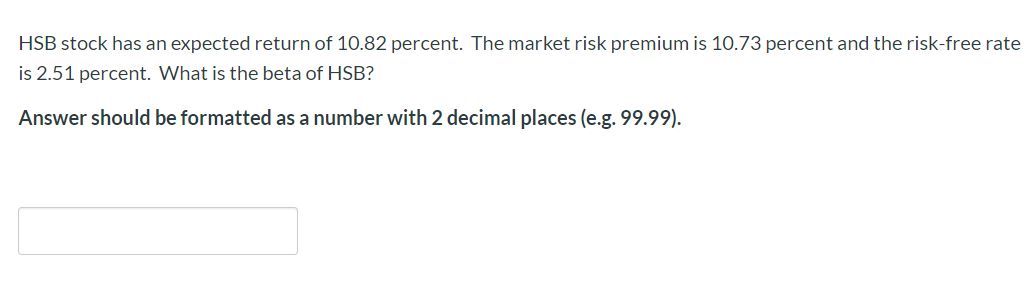Question

# The common stock of Jensen Shipping has a premium of 7.65 percent. What is the market...

The common stock of Jensen Shipping has a premium of 7.65 percent. What is the market risk-premium if the company's beta is 0.85? What is expected return on the market if the risk-free return is 4.00 percent?

A. Risk premium is 9.50 percent; Market expected return is 5.50 percent

B. Risk premium is 9.75 percent; Market expected return is 5.75 percent

C. Risk premium is 8.70 percent; Market expected return is 4.70 percent

D. Risk premium is 6.502 percent; Market expected return is 10.502 percent

E. Risk premium is 9.00 percent; Market expected return is 13.00 percent

Market risk premium = expected market return - risk-free rate

Expected market return = market risk premium + risk-free rate = 6.502% + 4% = 10.502%

#### Earn Coins

Coins can be redeemed for fabulous gifts.

Similar Homework Help Questions
• ### The common stock of Jensen Shipping has an expected return of 17.10 percent. The return on...

The common stock of Jensen Shipping has an expected return of 17.10 percent. The return on the market is 12 percent and the risk-free rate of return is 4.5. What is the beta of this stock? options: 1.26 1.68 0.75 1.41 1.52 A stock has an expected return of 12 percent, the risk-free rate is 5.4 percent, and the market risk premium is 5 percent. The beta of this stock must be options: 2.78 1.10 1.48 1.32 3.44

• ### The common stock of Jensen Shipping has an expected return of 12.08 percent. The return on...

The common stock of Jensen Shipping has an expected return of 12.08 percent. The return on the market is 9 percent and the risk-free rate of return is 3.5. What is the beta of this stock? 2.56 0.55 1.23 1.56 0.86

• ### 9. The common stock of Jensen Shipping has an expected return of 14.7 percent. The return...

9. The common stock of Jensen Shipping has an expected return of 14.7 percent. The return on the market is 10.8 percent and the risk-free rate of return is 3.8 percent. What is the beta of this stock? =1.56 -Please check the answers and show all work typed out. No excel or grid style please as I am on mobile.

• ### Cooley Company's stock has a beta of 0.60, the risk-free rate is 2.25%, and the market risk premium is 5.50%. What...Cooley Company's stock has a beta of 0.60, the risk-free rate is 2.25%, and the market risk premium is 5.50%. What is the firm's required rate of return? Do not round your intermediate calculations. 5.55% 4.38% 6.60% 6.16% 4.66%

• ### The risk-free rate of return is 2.8 percent and the market risk premium is 7.1 percent....

The risk-free rate of return is 2.8 percent and the market risk premium is 7.1 percent. What is the expected rate of return on a stock with a beta of 0.98?

• ### The risk-free rate of return is 2.5 percent, and the market risk premium is 11 percent....

The risk-free rate of return is 2.5 percent, and the market risk premium is 11 percent. What is the expected rate of return on a stock with a beta of 1.8?

• ### The risk-free rate of return is 3.68 percent and the market risk premium is 7.84 percent....

The risk-free rate of return is 3.68 percent and the market risk premium is 7.84 percent. What is the expected rate of return on a stock with a beta of 1.32?

• ### Sheridan Industries common stock has a beta of 1.2. If the market risk-free rate is 5.2...Sheridan Industries common stock has a beta of 1.2. If the market risk-free rate is 5.2 percent and the expected return on the market is 8.2 percent, what is Sheridan’s cost of common stock? I solved this problem incorrectly by doing Kes=Rrf+(Betaesx market risk premium) Kes=0.052+(1.2x0.082) Kes=0.052+0.098400 Kes=0.1504=15.0% I'm not sure what I am doing wrong. Please show full calculation. X Your answer is incorrect. Sheridan Industries common stock has a beta of 1.2. If the market risk-free rate is...

• ### Q11 - HSB stock has an expected return of 10.82 percent. The market risk premium is 10.73 percent and the risk-free rat...Q11 - HSB stock has an expected return of 10.82 percent. The market risk premium is 10.73 percent and the risk-free rate is 2.51 percent. What is the beta of HSB? Answer should be formatted as a number with 2 decimal places (e.g. 99.99).

• ### The risk-free rate of return is 2.5 percent, and the market risk premium is 11 percent....

The risk-free rate of return is 2.5 percent, and the market risk premium is 11 percent. What is the expected rate of return on a stock with a beta of 1.8? Group of answer choices 23.7 22.3 14.7 19.1